# scapGNN: Graph Neural Network-Based Framework for Single Cell Active Pathways and Gene Modules Analysis

#### 2023-08-08

library(scapGNN)

# Introduction

With the widespread availability of single-cell sequencing technology, numerous single-cell RNA-seq and ATAC-seq data are collected into public databases. However, the single-cell data have characteristics such as drop-out events and low library sizes. How to leverage these data to parse cellular heterogeneity at the pathway level and identify activated gene modules with multiple cell phenotypes is still a challenge.

Here, we propose scapGNN, an accurate, effective, and robust framework to comprehensively parse single-cell data at the pathway level from single-omics data or multi-omics integration data. The scapGNN model takes single-cell gene expression profiles or the gene activity matrix of scATAC-seq as input data. As shown in Figure 1A, the computing architecture consists of three modules:

1. A depp neural network-based autoencoder, which is used to extract cellular features, gene features, and mine latent associations between genes and cells.

2. A graph convolutional network-based graph autoencoder, which is used to construct cell-cell association network and gene-gene association network.

3. Random walk with restart (RWR), which is used to calculate the activity score of a pathway or gene set in each cell.

For the SNARE-seq dataset, scapGNN can integrate gene-cell association networks from scATAC-seq and scRNA-seq into one combined network by the Brown’s extension of the Fisher’s combined probability test. According to the combined gene-cell association network, pathway activity scores or activated gene modules are supported by single-cell multi-omics (Figure 2). scapGNN also designs abundant visualization programs to clearly display the analysis results. Taken together, its capabilities enable scapGNN to calculate pathway activity scores in a single cell, assess pathway heterogeneity between cells, identify key active genes in pathway, mine gene modules under multiple cell phenotypes, and provide gene and cell network data (Figure 1B).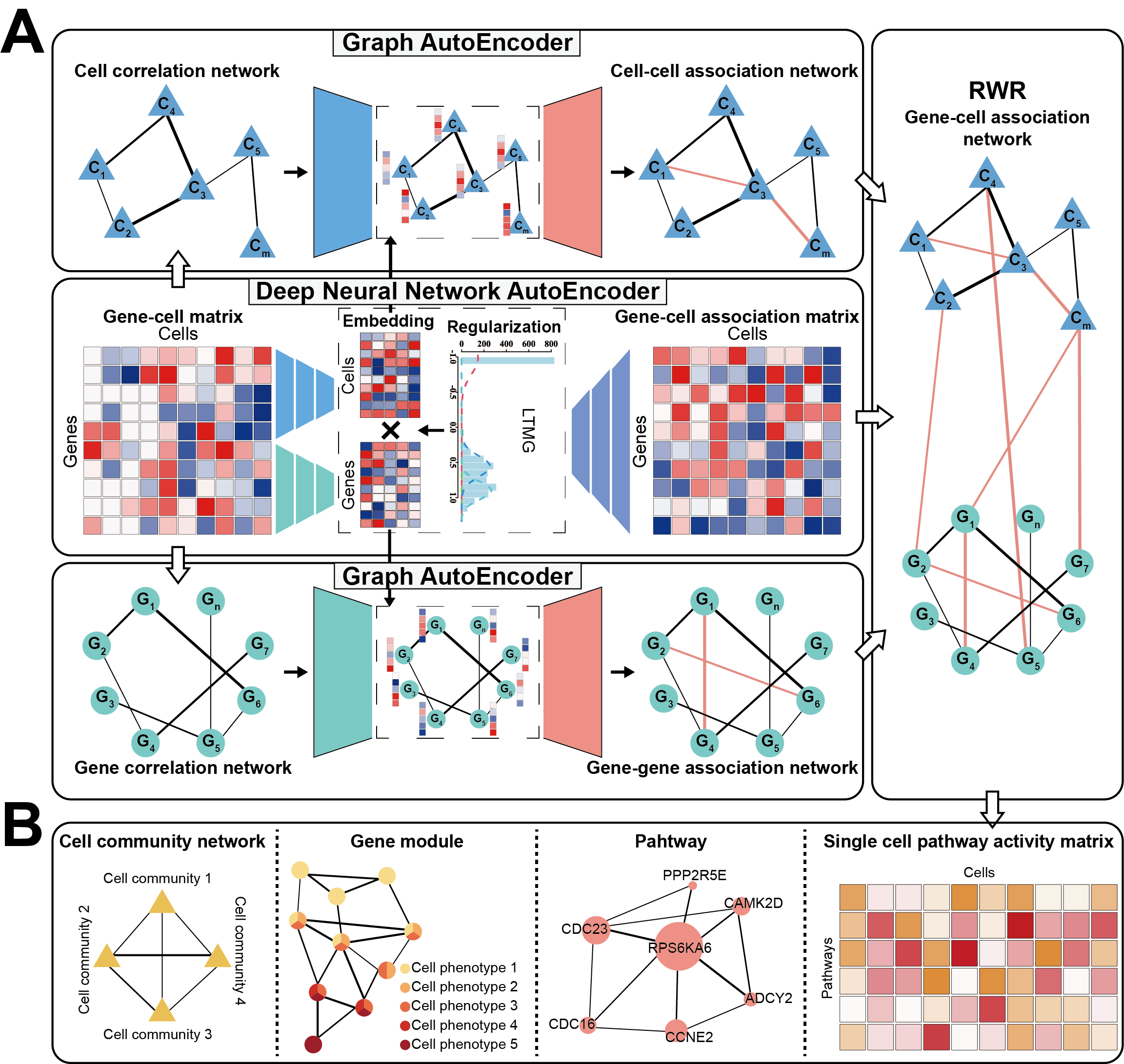Figure 1 Overview of the scapGNN framework.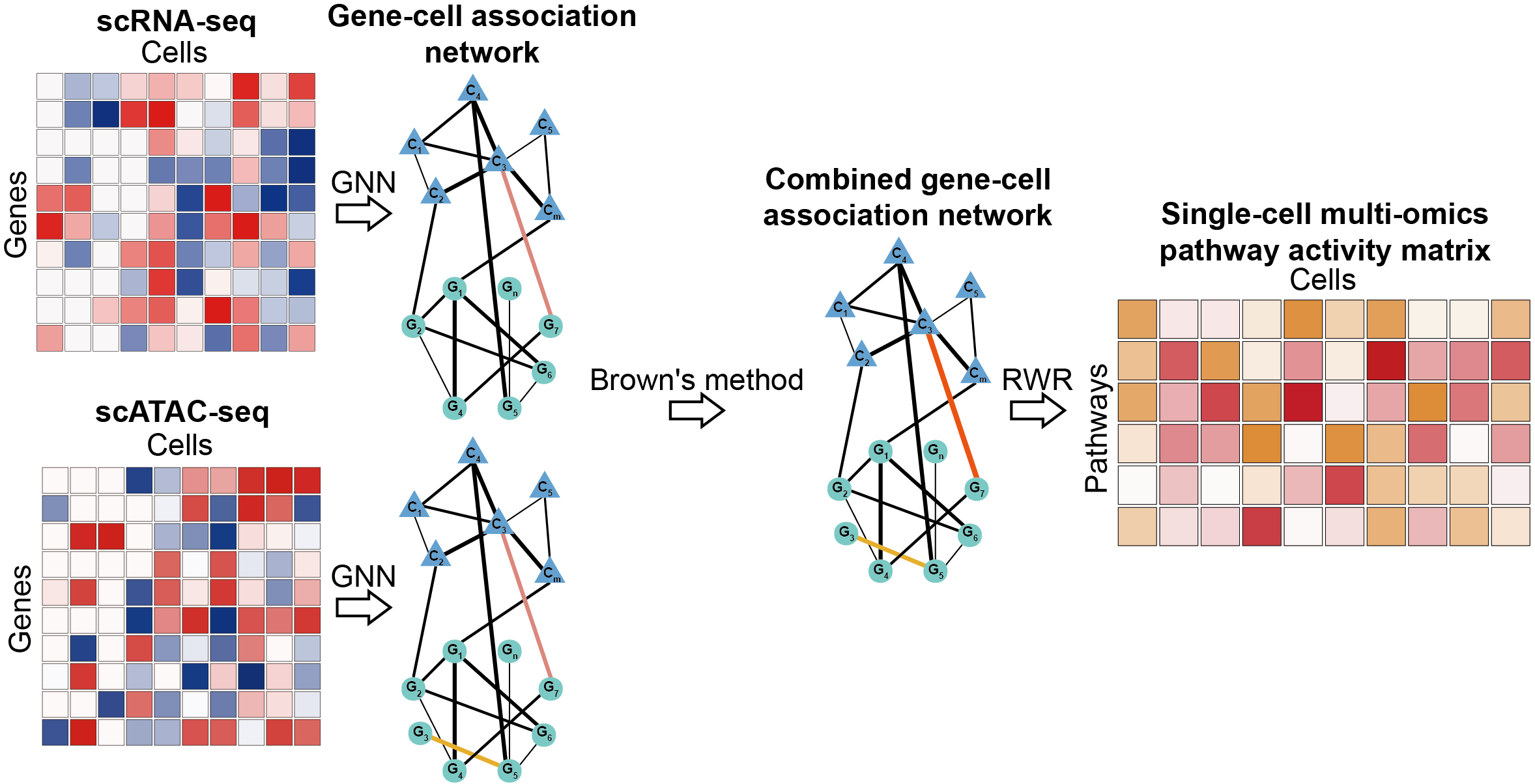Figure 2 The workflow of integrating scRNA-seq data and scATAC-seq data by scapGNN.

# Data preprocessing

## single-cell RNA-seq data

Single-cell gene expression profiles data needs to pass Seurat’s pre-processing workflow. These represent the selection and filtration of cells based on QC metrics, data normalization, and the detection of highly variable features.

library(Seurat)

# Load the PBMC dataset (Case data for seurat)

# Our recommended data filtering is that only genes expressed as non-zero in more than
# 1% of cells, and cells expressed as non-zero in more than 1% of genes are kept.
pbmc <- CreateSeuratObject(counts = pbmc.data, project = "case",
min.cells = 3, min.features = 200)

pbmc[["percent.mt"]] <- PercentageFeatureSet(pbmc, pattern = "^MT-")

pbmc <- subset(pbmc, subset = nFeature_RNA > 200 & nFeature_RNA < 2500 & percent.mt < 5)

# Normalizing the data.
pbmc <- NormalizeData(pbmc, normalization.method = "LogNormalize")

# Identification of highly variable features.
pbmc <- FindVariableFeatures(pbmc, selection.method = 'vst', nfeatures = 2000)

# Run Preprocessing() of scapGNN.
Prep_data <- Preprocessing(pbmc,verbose=FALSE)

Detailed procedures and descriptions can be found in vignettes of Seurat (https://satijalab.org/seurat/).

# Users can also directly input data in a data frame or matrix format which contains hypervariable genes and is log-transformed.
data("Hv_exp")
Prep_data <- Preprocessing(Hv_exp,verbose=FALSE)
summary(Prep_data)
#>               Length Class  Mode
#> orig_dara      45000 -none- numeric
#> cell_features  45000 -none- numeric
#> gene_features  45000 -none- numeric
#> ltmg_matrix        1 -none- function
#> gene_adj      250000 -none- numeric

## single-cell ATAC-seq data

First, the Signac package is used to quantify the activity of each gene in the genome by assessing the chromatin accessibility associated with each gene, and create a new gene activity matrix derived from the scATAC-seq data. For details, please refer to vignettes of Signac (https://satijalab.org/signac/index.html). As with single-cell gene expression profiles, the ensuing gene activity matrix is normalized and detected highly variable features by the Seurat package. Finally, the Preprocessing() of scapGNN further processes the data into the required format.

# Construct gene-cell association network

Using the Preprocessing() function results of scRNA-seq or scATAC-seq as input, the ConNetGNN() function evaluates whether gene-gene, cell-cell, and gene-cell are associated and levels of strength. Finally, an undirected and weighted gene-cell network is constructed and use for subsequent analysis.

The ConNetGNN() function is implemented based on pytorch, so an appropriate python environment is required:

• python >=3.9.7
• pytorch >=1.10.0 (CPU)
• sklearn >=0.0
• scipy >=1.7.3
• numpy >=1.19.5

We also provide environment files for conda: /inst/extdata/scapGNN_env.yaml. Users can install it with the command: conda env create -f scapGNN_env.yaml.

library(coop)
library(reticulate)
library(parallel)
# Data preprocessing
data("Hv_exp")
Prep_data <- Preprocessing(Hv_exp)

# Run ConNetGNN()
ConNetGNN_data <- ConNetGNN(Prep_data,python.path="../miniconda3/envs/scapGNN_env/python.exe")

# View the content of the ConNetGNN() results.
data(ConNetGNN_data)
summary(ConNetGNN_data)
#>                   Length Class  Mode
#> cell_network        8100 -none- numeric
#> gene_network      250000 -none- numeric
#> gene_cell_network  45000 -none- numeric

For the SNARE-seq dataset, the ConNetGNN() results of scRNA-seq and scATAC-seq can be integrated into a combined gene-cell association network via InteNet().

library(ActivePathways)
library(parallel)
data(RNA_net)
data(ATAC_net)
RNA_ATAC_IntNet<-InteNet(RNA_net,ATAC_net,parallel.cores=1)


# Infer pathway activity score matrix at the single-cell level

For the gene-cell association network constructed by ConNetGNN(), the scPathway() function uses RWR algorithm to calculate the pathway activity score of each cell.

library(parallel)
library(utils)
# Load the result of the ConNetGNN function.
data(ConNetGNN_data)
# We recommend the use of a compiler.
# The compiler package can be used to speed up the operation.
# library(compiler)
# scPathway<- cmpfun(scPathway)
scPathway_data<-scPathway(ConNetGNN_data,gmt.path=system.file("extdata", "KEGG_human.gmt", package = "scapGNN"),parallel.cores=1)

The result scPathway_data of scPathway() is a matrix of pathway activity scores, with rows as pathways and columns as cells.

data(scPathway_data)
scPathway_data[1:3,1:3]
#>                                        H9_0h_1 H9_0h_2 H9_0h_3
#> Ras signaling pathway [PATH:hsa04014]     0.20    0.19    0.17
#> Rap1 signaling pathway [PATH:hsa04015]    0.08    0.10    0.10
#> MAPK signaling pathway [PATH:hsa04010]    0.68    0.59    0.70
library(pheatmap)
pheatmap(scPathway_data)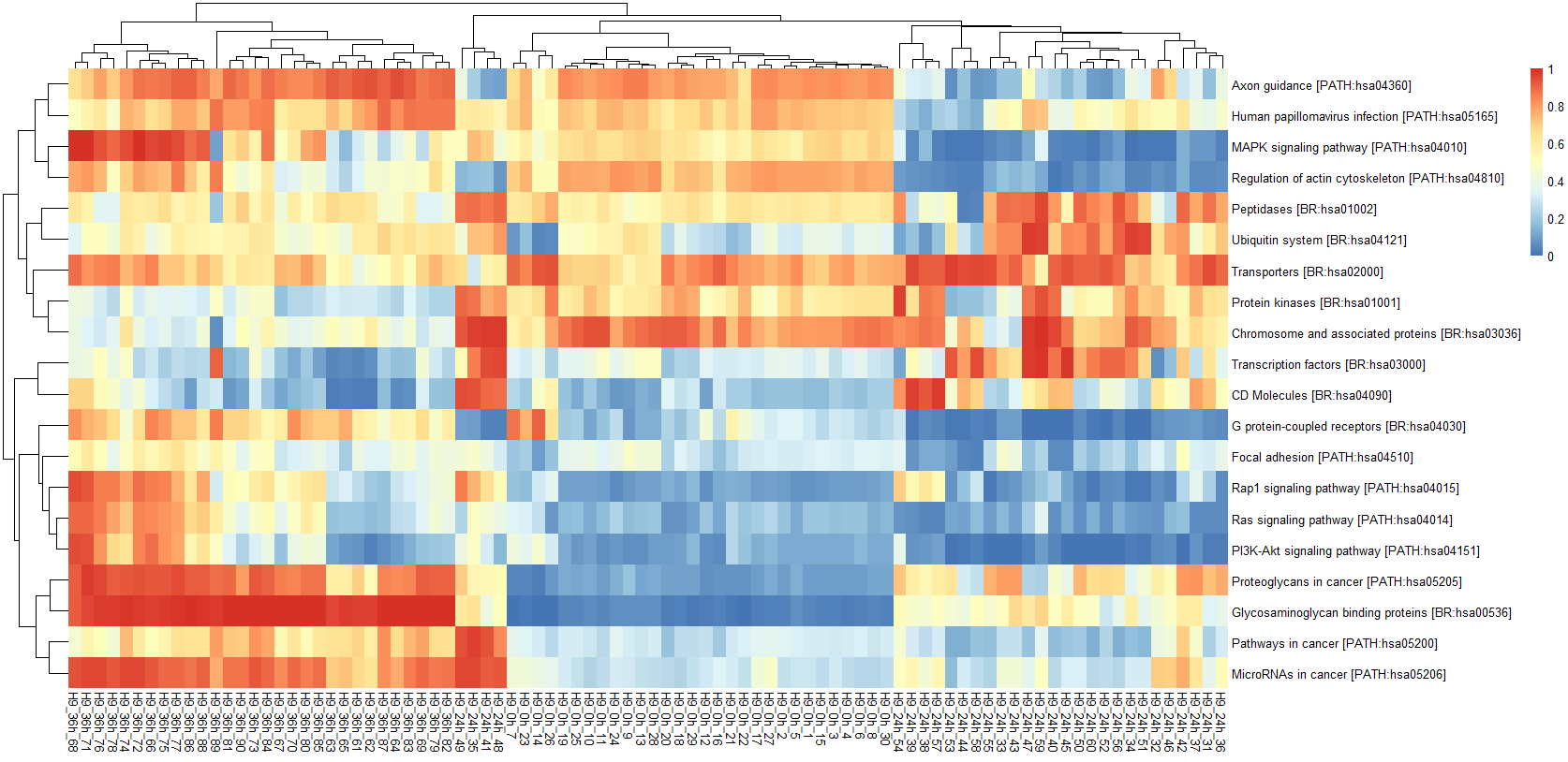Use the plotGANetwork() function to plot the gene network of pathways in the specified cell phenotype.

library(igraph)
#>
#> Attaching package: 'igraph'
#> The following objects are masked from 'package:stats':
#>
#>     decompose, spectrum
#> The following object is masked from 'package:base':
#>
#>     union

data(ConNetGNN_data)
data("Hv_exp")

# Construct cell set.
index<-grep("0h",colnames(Hv_exp))
cellset<-colnames(Hv_exp)[index]

# Construct gene set.
geneset<-pathways[[which(names(pathways)=="Tight junction [PATH:hsa04530]")]]

plotGANetwork(ConNetGNN_data,cellset,geneset,vertex.label.dist=1.5,main = "Tight junction [PATH:hsa04530]")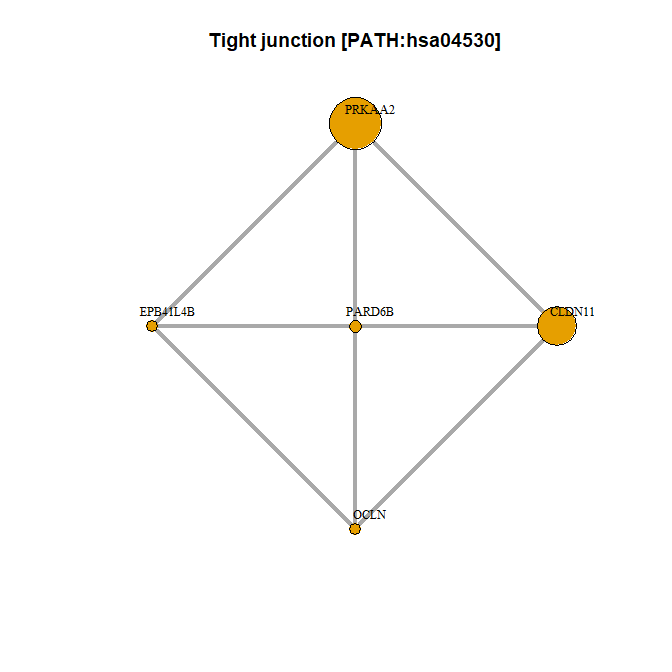The plotGANetwork() function can construct and display a cell community network.

require(igraph)
require(graphics)

data(ConNetGNN_data)

# Construct the cell phenotype vector.
cell_id<-colnames(ConNetGNN_data[["cell_network"]])
temp<-unlist(strsplit(cell_id,"_"))
cell_phen<-temp[seq(2,length(temp)-1,by=3)]
names(cell_id)<-cell_phen
#>        0h        0h        0h        0h        0h        0h
#> "H9_0h_1" "H9_0h_2" "H9_0h_3" "H9_0h_4" "H9_0h_5" "H9_0h_6"
plotCCNetwork(ConNetGNN_data,cell_id,edge.width=10)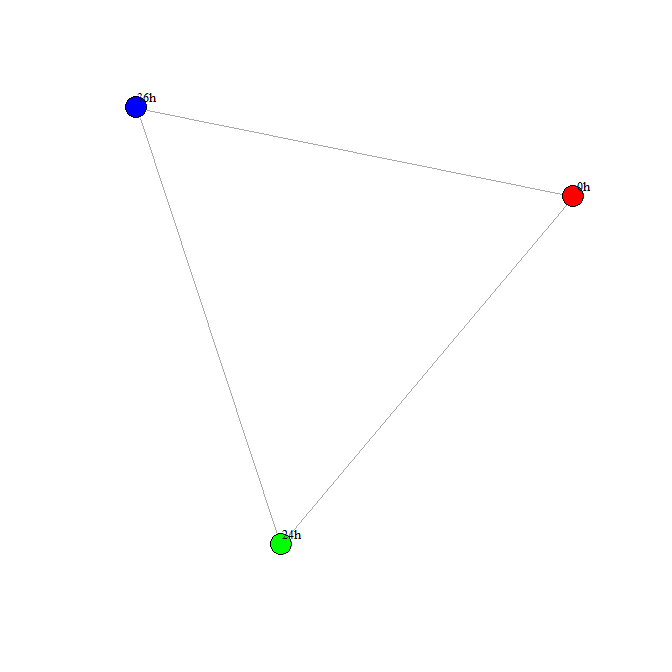# Identification of activated gene modules

The cpGModule() can identify cell phenotype activated gene module.

require(parallel)
require(stats)

# Load the result of the ConNetGNN function.
data(ConNetGNN_data)
data(Hv_exp)

# Construct the cell set corresponding to 0h.
index<-grep("0h",colnames(Hv_exp))
cellset<-colnames(Hv_exp)[index]
H9_0h_cpGM_data<-cpGModule(ConNetGNN_data,cellset,parallel.cores=1)
#> Start RWR
#> Start perturbation analysis
summary(H9_0h_cpGM_data)
#>     Genes                 AS               Pvalue               FDR
#>  Length:30          Min.   :0.001181   Min.   :0.000e+00   Min.   :0.000e+00
#>  Class :character   1st Qu.:0.001317   1st Qu.:3.630e-07   1st Qu.:2.018e-05
#>  Mode  :character   Median :0.001513   Median :4.551e-05   Median :1.422e-03
#>                     Mean   :0.001656   Mean   :4.345e-04   Mean   :8.280e-03
#>                     3rd Qu.:0.001815   3rd Qu.:6.034e-04   3rd Qu.:1.325e-02
#>                     Max.   :0.002608   Max.   :2.830e-03   Max.   :4.717e-02

Use the plotGANetwork() function to plot the gene network of module.

library(igraph)

data(ConNetGNN_data)
data("Hv_exp")
data("H9_0h_cpGM_data")

# Construct cell set.
index<-grep("0h",colnames(Hv_exp))
cellset<-colnames(Hv_exp)[index]

# Construct gene set.
geneset<-H9_0h_cpGM_data\$Genes

plotGANetwork(ConNetGNN_data,cellset,geneset,vertex.label.dist=1.5,main = "Gene network of 0h cells activated gene module")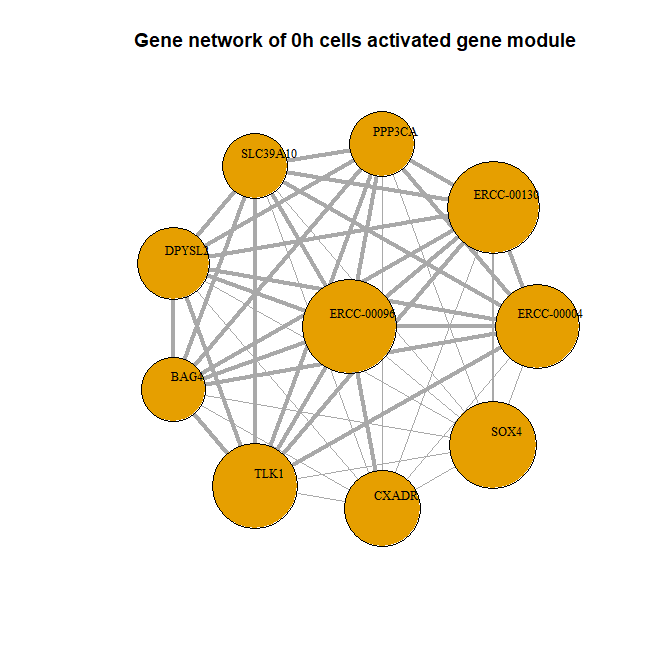For multiple cellular phenotypes (e.g. classification, time stage, etc.), cpGModule() identifies the activated gene modules individually. The plotMulPhenGM() function then quantifies the activation strength of genes in different cell phenotypes and displays gene networks under multiple cell phenotypes.

require(igraph)
require(grDevices)
# Load the result of the ConNetGNN function.
data(ConNetGNN_data)
# Obtain cpGModule results for each cell phenotype.
data(H9_0h_cpGM_data)
data(H9_24h_cpGM_data)
data(H9_36h_cpGM_data)
data.list<-list(H9_0h=H9_0h_cpGM_data,H9_24h=H9_24h_cpGM_data,H9_36h=H9_36h_cpGM_data)
plotMulPhenGM(data.list,ConNetGNN_data,margin=-0.05)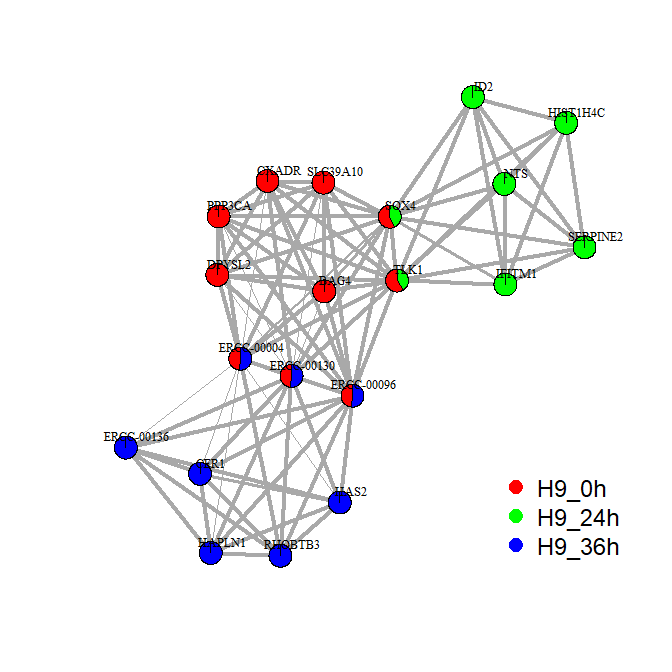# Set plotgraph=F to get the network data.
data_graph<- plotMulPhenGM(data.list,ConNetGNN_data,margin=-0.05,plotgraph=F)
#Obtain the adjacency matrix of the network by the method of igraph package.
# The value of Gene_adj_matrix is the strength of the gene-gene association.

# Data analysis and visualization combined with Seurat package

The single-cell pathway activity matrix can be docked with the Seurat package to perform non-linear dimensional reduction, identify differentially active pathways, draw heat map, violin plot, and more.

library(dplyr)
library(Seurat)
library(patchwork)

data(scPathway_data)
data <- CreateSeuratObject(counts = scPathway_data, project = "Pathway")
all.genes <- rownames(data)
data <- ScaleData(data, features = all.genes,verbose = FALSE)
data <- RunPCA(data, features =all.genes,verbose = FALSE)
data <- FindNeighbors(data, verbose = FALSE)
data <- FindClusters(data, resolution = 0.5,verbose = FALSE)
data <- RunUMAP(data,dims = 1:15, verbose = FALSE)

cell_phen<-unlist(strsplit(colnames(scPathway_data),"_"))
cell_phen<-cell_phen[seq(2,length(cell_phen)-1,by=3)]

data@active.ident<-as.factor(cell_phen)

# Visualize non-linear dimensional reduction
DimPlot(data, reduction = "umap")

# Identify differentially active pathways
diff.pathways <- FindAllMarkers(data,verbose = FALSE)

# violin plot
VlnPlot(data, features = "MAPK signaling pathway [PATH:hsa04010]")

# heat map
DoHeatmap(data, features = row.names(scPathway_data))